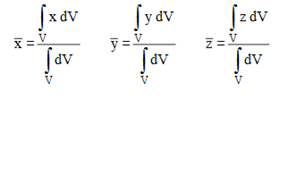Centroid of volumes can be determined similar to the centroid of lines. Suppose the value of moment is zero at a point, it is the centroidal point of that figure.

For this condition, the value of distance of the point from the axes in three dimensions is considered for determining the integral. The choice of one, two or three integral formulation is used depending upon the differential volume of the figure.

Suppose the elementary mass and volume be dm and dV then the equations of integral can be given by –

For three-dimensional figure, the ratio of integrals are written in the following manner-Links of Next Mechanical Engineering Topics:-### Customer Reviews

My Homework Help
Rated 5.0 out of 5 based on 510 customer reviews at